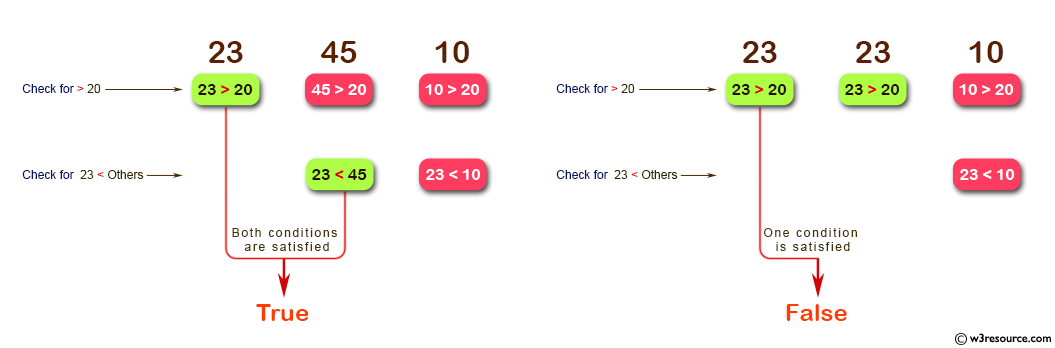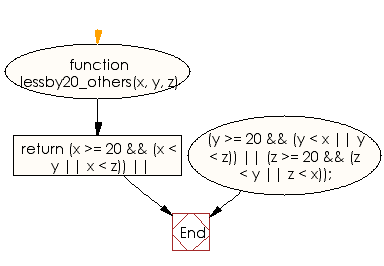# JavaScript: Check from three given integers that whether a number is greater than or equal to 20 and less than one of the others

## JavaScript Basic: Exercise-44 with Solution

Write a JavaScript program to check from three given integers that whether a number is greater than or equal to 20 and less than one of the others.

Pictorial Presentation:Sample Solution:

HTML Code:

``````<!DOCTYPE html>
<html>
<meta charset="utf-8">
<meta name="viewport" content="width=device-width">
<title>JavaScript program to check from three given integers that whether a number is greater than or equal to 20 and less than one of the others.</title>
<body>

</body>
</html>
```
```

JavaScript Code:

``````function lessby20_others(x, y, z)
{
return (x >= 20 && (x < y || x < z)) ||
(y >= 20 && (y < x || y < z)) ||
(z >= 20 && (z < y || z < x));
}
console.log(lessby20_others(23, 45, 10));
console.log(lessby20_others(23, 23, 10));
console.log(lessby20_others(21, 66, 75));
```
```

Sample Output:

```true
false
true
```

Flowchart:Live Demo:

Improve this sample solution and post your code through Disqus

What is the difficulty level of this exercise?

Test your Programming skills with w3resource's quiz.

﻿

## JavaScript: Tips of the Day

Checks if a string is an anagram of another string (case-insensitive, ignores spaces, punctuation and special characters)

Example:

```const isAnagram = (str1, str2) => {
const normalize = str =>
str
.toLowerCase()
.replace(/[^a-z0-9]/gi, '')
.split('')
.sort()
.join('');
return normalize(str1) === normalize(str2);
};
console.log(isAnagram('iceman', 'cinema')); // true
```

Output:

```true
```